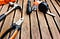Photo by Louis Hansel @shotsoflouis on Unsplash

# Sextic Polynomials — A Practical Approach

At a young age a builder taught me how to mark out a right angle using the 3,4,5 triangle rule with a tape measure. That math practicality has stuck with me and recently I have been working to decipher it into its ‘LEGO block’ constituents to increase intuitive understanding.

This post adopts a ‘Tool Kit’ of methods of finding polynomial roots using basic math including Similar Triangles, ‘internal Quadratic architecture’ and formula adaptations and creation of twin function ‘SOSO’ nodes as application points for root approximation methods. Its purpose is to foster intuitive thinking over convenience solutions.

The intention isn’t…

# Cubic Polynomials — Managing the Architecture to Calculate Roots

## Rotating cubic roots into managed quadratic segments where the math is easier!

This post presents a novel method for approximating 1st roots of cubic polynomials that avoids the lengthy gradient and height calculation iterations associated with Newton’s and some of my own previously posted methods.

It particularly addresses the task of approximating cubic polynomial roots in ‘difficult to get to’ locations - namely where root gradients are low and close to turning points. Such ‘architecture’ would normally require two or more iterations to solve.

This method exploits the amazing symmetry of cubic polynomials and their ‘component architecture’ by ‘rotating’ roots into segments where the underlying quadratic architecture approximates the cubic curvature.

This…

# Cubic Polynomials – A Simpler Approach

## An intuitive way to find the 2nd and 3rd roots

While we are all familiar with finding the roots of a quadratic using the Quadratic Equation, it can be complex to find the roots of a higher order polynomial.

We typically need to find one factor by brute force, then divide through to create a quadratic.

I’ve been working on a simpler approach that I’m sharing in this post.

My goal is to make the process a lot simpler by eliminating polynomial long division.

This approach assumes knowledge of algebra and introductory calculus (differentiating polynomials) at the high school level.

I hope it helps you to think in a fresh…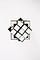Photo by Honey Yanibel Minaya Cruz on Unsplash

# Quartic Polynomials -From Square Roots to Roots

## Using Square Roots - with ‘Division by Vision’ to find Quartic Roots

This post introduces a ‘Roots to Roots’ approximation by using a specific Square Root of the underlying Polynomial ‘Architecture’. The math is simplified with use of a previously posted division method, Polynomials — Division by Vision, which covers polynomial division without remainders.

We are all familiar with ‘Perfect Square Quadratics’ that can be factored as perfect squares, such as the square of a linear factor, (x+2)²=x²+4x+4. While the typical Quadratic isn’t ‘perfect’, we can turn it into one by the familiar method of ‘completing the square’, i.e. by adding a constant number.

‘Imperfect’ Quartic Polynomials can similarly be converted to…

# Deriving the Quadratic Equation From the Roots Up

## Solving Quadratics using gradients at the roots

An important characteristic of the Quadratic Polynomial y=ax²+bx+c is that it retains its parabolic ax² shape and size wherever it is located in the x-y grid. The other terms, bx +c are just the, “Sit where we say”, ushers!

This architectural symmetry and stability means that the gradients at the roots are of equal magnitude and opposite sign and can be used to calculate the roots.

For example, we will show how a quadratic such as y=2x²+x-3 has root gradients: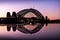Photo by Susan Kuriakose on Unsplash

# The Simplest Cubic Root

## A very simple Cubic polynomial root approximation using architectural symmetry and similar triangles

The architectural symmetry of Cubic polynomials supports many root approximation methods, helping intuitive understanding and with a little work, can be transposed to higher orders to minimise more complex math. This post expands on an earlier post, Cubic Polynomials — Using Similar Triangles to Approximate Roots, which promoted the use of Similar Triangles derived from Turning and Inflection Points.

Both methods are very simple and it is difficult to quantify pros and cons in a short post. The latter, being very easily formularised and not requiring calculus beyond finding the Inflection Point Ip is the quicker and simpler of the…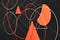Photo by Martin Sanchez on Unsplash

# Cubic Polynomials - Using Similar Triangles to Approximate Roots

## Applying Similar Triangles to approximate roots aided by ‘natural’ Cubic architecture

While similar triangles can be used with polynomials of any order, this introductory post is presented in cubics to exploit the compliant architecture while highlighting the practicality and simplicity of similar triangle math.

The method has particular advantages in higher order polynomials when supported by various changeable architectures, as it can alleviate onerous iterations of gradient and height calculations. This will be the subject of another post.

This post assumes math at high school level.

## Similar Triangles

Similar triangles strike a chord across the x axis between points or nodes being line intercepts on the function.

The closer the nodes are together…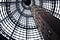Melbourne Shot Tower Photo by Alex Knight on Unsplash

# Quintic Polynomials — Finding Roots From Primary and Secondary Nodes; a Double Shot!

## Using twin function proximal nodes for application of Newton’s Approximation to find roots

While Newton’s approximation is universal, its accuracy largely depends on having application nodes proximal to roots to eliminate the need for iterations. It is also impacted by the steepness of the gradient at the root and the variability of the function’s root gradient with the node tangent. To improve options for node selection I recently posted, Quartic Polynomials-Calculate Roots Using Twin Function Nodes, which uses the architecture and symmetry of polynomials to find roots using ‘SOSO’; a confected twin function with Same Opposite Same Opposite +/- coefficients.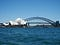Courtesy Icepoint Unsplash

# Quartic Polynomials — Calculate Roots Using Twin Function Nodes

## Exploiting architectural symmetry of Quartic Polynomials to calculate roots using Twin Function Nodes created by SOSO

This post demonstrates the application to Quartic polynomials of SOSO which is a confected twin function with Same Opposite Same Opposite +/- coefficients. It creates strategic node intercepts, for the application of Newton’s or other approximation methods where applicable.

I recently posted Polynomial Roots — ‘SOSO’ Easy with Curved Segment transplants, using the ‘architecture’ and symmetry of Cubic Polynomials to find roots by curved Segment Transplants Incorporating Node gradients or ‘STING’ as an alternative to Newton’s straight line approximation.

My focus here is on 1st and 2nd roots by SOSO, as I have previously presented a modified Quadratic Equation for…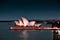Sydney Opera House-SOSO curves with a million fitted tiles! Photo by Arvin Wiyono on Unsplash

# Polynomial Roots — Applying Curved Segment transplants from ‘SOSO’ Nodes

## Using the ‘architecture’ to find roots from confected ‘SOSO’ nodes with transplanted segments having the same initial gradients as the recipient root segments.

Since I presented Cubic Polynomials — A Simpler Approach to find the last two roots of any polynomial given the other roots, my focus has been on improving node coordinates for application of Newton’s Approximation in finding first roots.

While exact lengthy numerical methods and calculators are of course available, I prefer to use ‘visual architecture’ as it aids learning, while also finding good approximate solutions. My objective in this post is twofold:

• to simplify and improve initial node coordinates by confecting twin Same Opposite Same Opposite, or ‘SOSO’ functions with intercepts, and;
• to replace Newton’s straight line hypotenuse approximations…## Greg Oliver

I’m passionate about maths and making it simpler to enjoy. Retired Engineer currently writing maths book at a high school level.